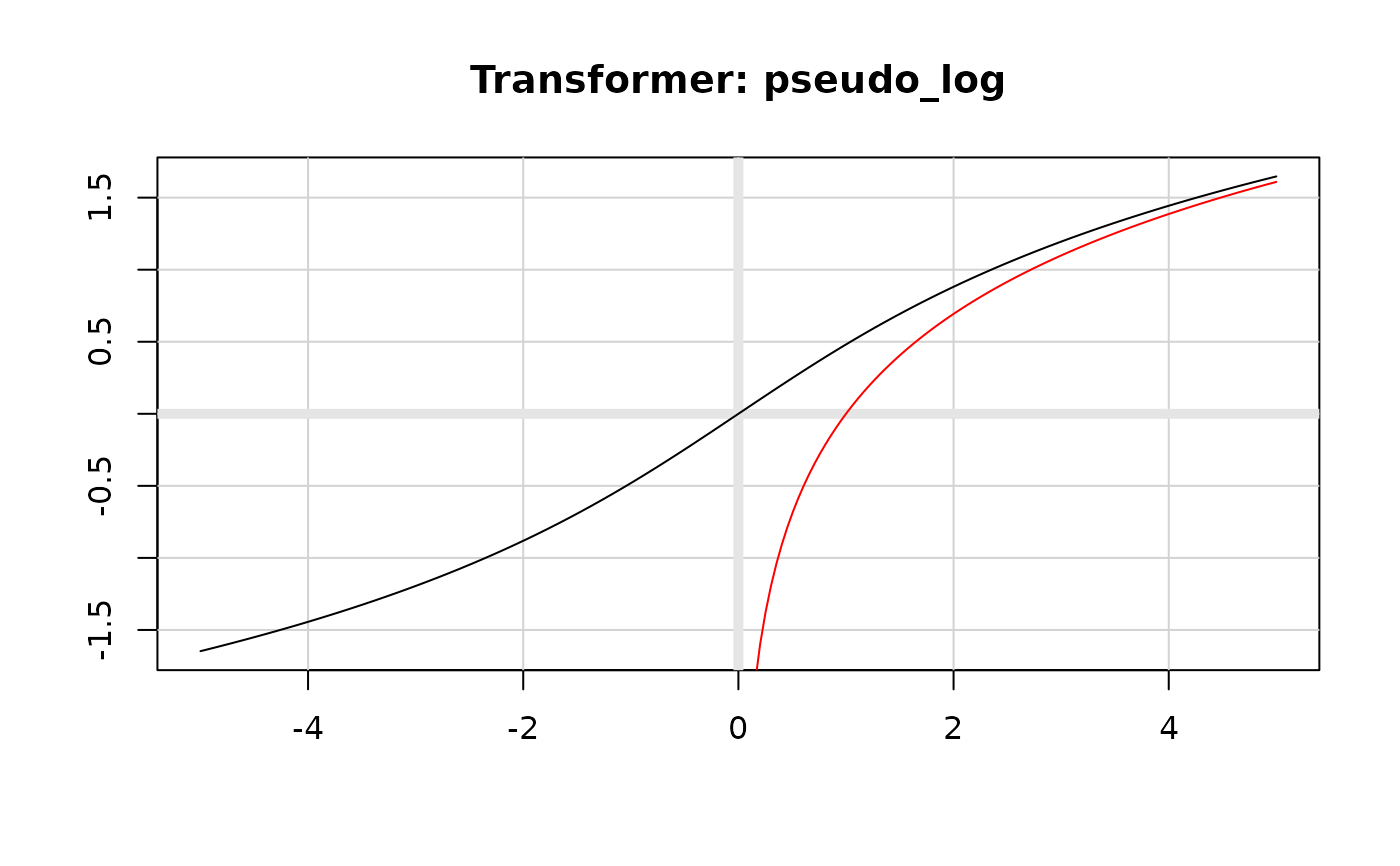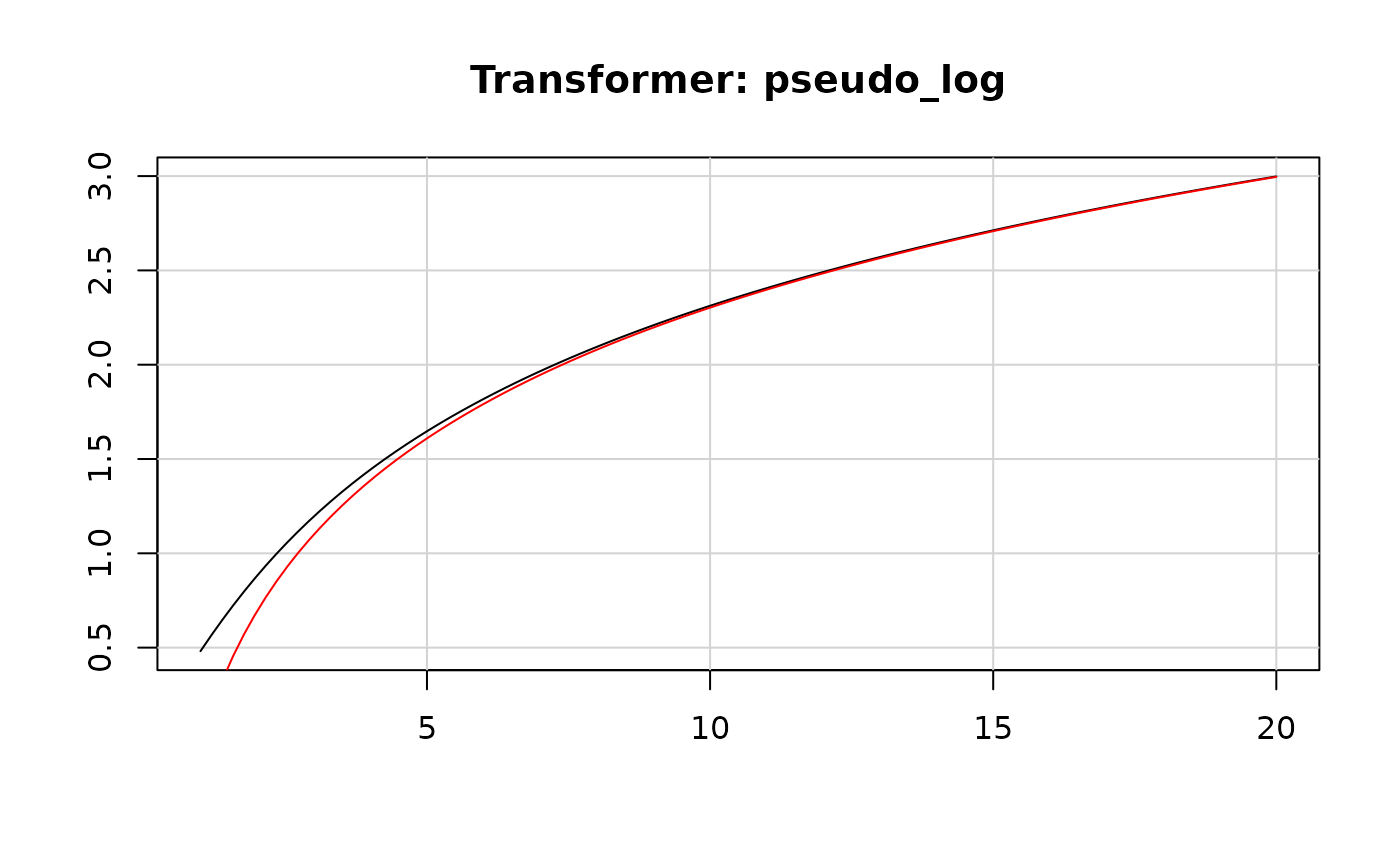• log_trans(): log(x)

• log1p(): log(x + 1)

• pseudo_log_trans(): smoothly transition to linear scale around 0.

## Usage

log_trans(base = exp(1))

log10_trans()

log2_trans()

log1p_trans()

pseudo_log_trans(sigma = 1, base = exp(1))

## Arguments

base

base of logarithm

sigma

Scaling factor for the linear part of pseudo-log transformation.

## Examples

plot(log2_trans(), xlim = c(0, 5))plot(log_trans(), xlim = c(0, 5))plot(log10_trans(), xlim = c(0, 5))plot(log_trans(), xlim = c(0, 2))plot(log1p_trans(), xlim = c(-1, 1))# The pseudo-log is defined for all real numbers
plot(pseudo_log_trans(), xlim = c(-5, 5))
lines(log_trans(), xlim = c(0, 5), col = "red")# For large positives nubmers it's very close to log
plot(pseudo_log_trans(), xlim = c(1, 20))
lines(log_trans(), xlim = c(1, 20), col = "red")